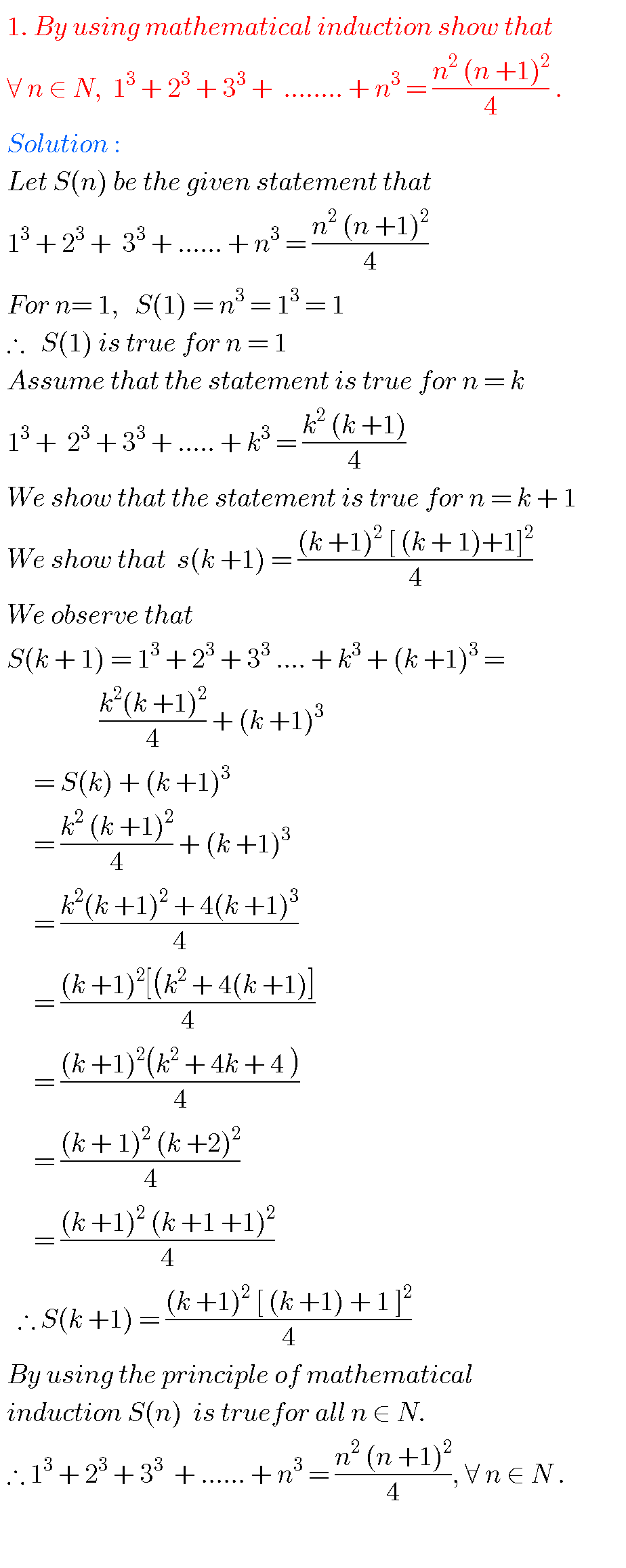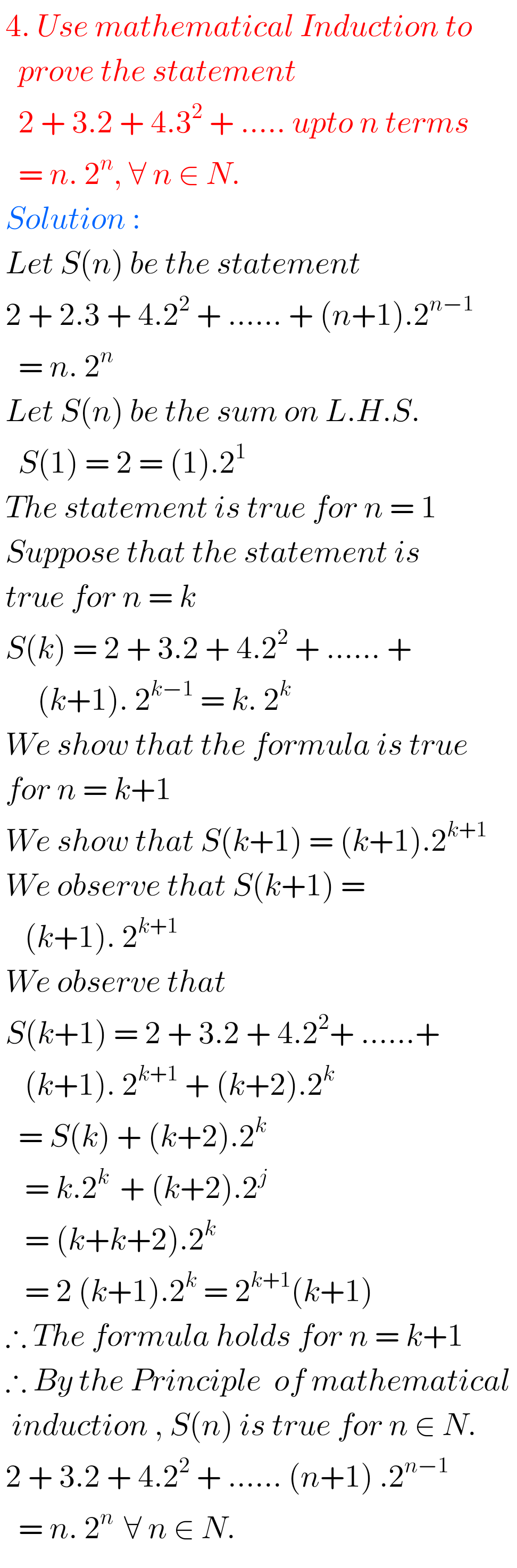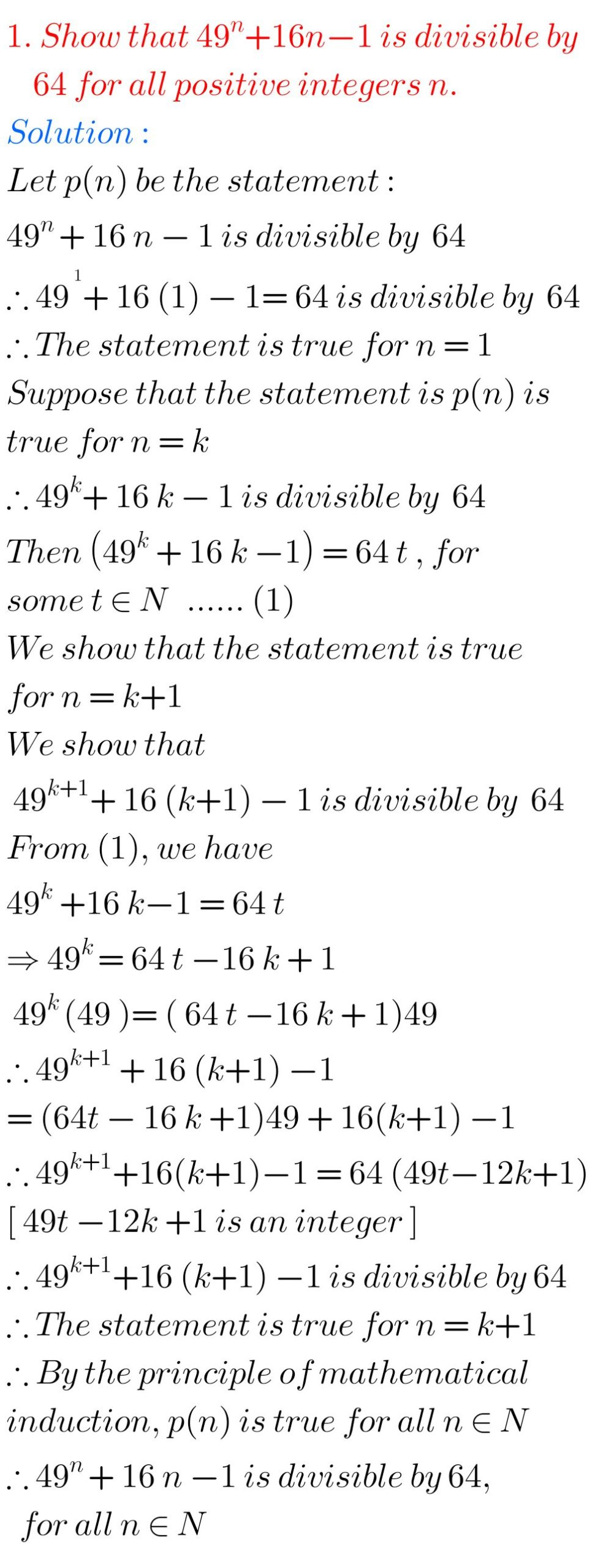# MATHEMATICAL INDUCTION, INTERMEDIATE FIRST YEAR PROBLEMS WITH SOLUTIONS

Mathematics Intermediate first year 1A Mathematical Induction solutions for some problems.

These solutions are very simple to understand.

Study the textbook lesson Mathematical Induction very well

Observe the example problems and solutions given in the textbook

You can also see

Inter Maths 1A textbook solutions

Inter Maths 1B textbook solutions

Inter Maths IIA textbook solutions

Inter Maths IIB textbook solutions

Nios solutions for maths 311 book 1 Sets 1.1

M

## Mathematical induction solutions

Chapter 2

Mathematical Induction

Exercise 2(a)

You can see solutions in pdf

Click below for solutions of Mathematical Induction

Using-mathematical-induction

## MATHEMATICAL INDUCTION SOLUTIONS INTERMEDIATEYou can also see

Note: Observe the solutions of mathematical induction and try them in your own methods.

Some more solutionsYou can see the solutions for junior inter maths 1A for examination purpose.

2. Matrices

Ncert solutions for maths class 6 th some chapters solutions

Ncert solutions for maths class 7 th some chapters solutions

Ncert solutions for maths class 8 th some chapters solutions

Nceet solutions for class 8 chapter 12

Inter maths 1a solutions

Inter maths trigonometry solutions

Maths real numbers solutions class 10

### 7 thoughts on “MATHEMATICAL INDUCTION, Intermediate 1st year problems with solutions,solutions for Mathematical Induction Inter first year 1A ”

1.good

2.Thanks buddy

3.Good work keep it up.
Ur doing a great job

4.Nice Tq

5.Thanks

1.Doing great job

6.Super solution boss